### Elina Azrilyan

October 25th, 2019

Exercise 11 The price of one share of stock in the Pilsdorff Beer Company (see Exercise 8.2.12) is given by Yn on the nth day of the year. Finn observes that the differences Xn = Yn+1 − Yn appear to be independent random variables with a common distribution having mean μ = 0 and variance σ2 = 1/4. If Y1 = 100, estimate the probability that Y365 is

1. ≥ 100.
z <- (100 - 100)/(sqrt(365)*sqrt(1/4))
pnorm(z, lower.tail = FALSE)
##  0.5
1. ≥ 110.
z <- (110 - 100)/(sqrt(365)*sqrt(1/4))
pnorm(z, lower.tail = FALSE)
##  0.1475849
1. ≥ 120.
z <- (120 - 100)/(sqrt(365)*sqrt(1/4))
pnorm(z, lower.tail = FALSE)
##  0.01814355
1. Calculate the expected value and variance of the binomial distribution using the moment generating function.

The calculation for mgf for exponential distribution can be found here: https://newonlinecourses.science.psu.edu/stat414/node/73/

knitr::include_graphics("BinomMt.png")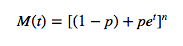We take the derivative of the mfg to get the expected value:

knitr::include_graphics("binomMtvar.png")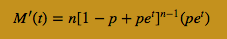for t=0 this simplifies to μ=n*p

We take the 2nd derivative to get the variance:

knitr::include_graphics("binomMtmean.png")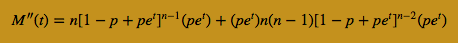for t=0 this simplifies to Variance = np(1 − p)

1. Calculate the expected value and variance of the exponential distribution using the moment generating function.

The calculation for mgf for exponential distribution can be found here:

https://www.statlect.com/fundamentals-of-probability/moment-generating-function

knitr::include_graphics("EVmgfExp.png")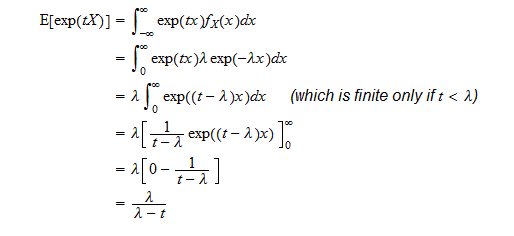We take the derivative of the mfg to get the expected value:

M′(t)=λ/(λ−t)^2

M′(0)=λ/(λ−0)^2 = λ/λ^2 = 1/λ

We take the 2nd derivative to get the variance:

knitr::include_graphics("varexp.png")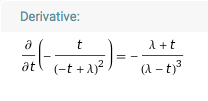M′′(0) = λ/λ^3 = 1/λ^2

Sources:

https://www.statlect.com/fundamentals-of-probability/moment-generating-function

https://newonlinecourses.science.psu.edu/stat414/node/73/